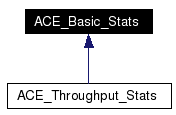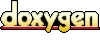# ACE_Basic_Stats Class Reference

Collect basic stats about a series of samples. More...

`#include <Basic_Stats.h>`

Inheritance diagram for ACE_Basic_Stats:[legend]
List of all members.

## Public Member Functions

ACE_Basic_Stats (void)
Constructor.

ACE_UINT32 samples_count (void) const
The number of samples received so far.

void sample (ACE_UINT64 value)
Record one sample.

void accumulate (const ACE_Basic_Stats &rhs)
Update the values to reflect the stats in rhs.

void dump_results (const ACE_TCHAR *msg, ACE_UINT32 scale_factor) const
Dump all the samples.

## Public Attributes

ACE_UINT32 samples_count_
The number of samples.

ACE_UINT64 min_
The minimum value.

ACE_UINT32 min_at_
The number of the sample that had the minimum value.

ACE_UINT64 max_
The maximum value.

ACE_UINT32 max_at_
The number of the sample that had the maximum value.

ACE_UINT64 sum_
The sum of all the values.

## Detailed Description

Collect basic stats about a series of samples.

Compute the average and standard deviation (aka jitter) for an arbitrary number of samples, using constant space. Normally used for latency statistics.

Definition at line 32 of file Basic_Stats.h.

## Constructor & Destructor Documentation

 ACE_BEGIN_VERSIONED_NAMESPACE_DECL ACE_INLINE ACE_Basic_Stats::ACE_Basic_Stats ( void )
 Constructor. The number of samples is pre-allocated, and cannot changes once the class is initialized. Definition at line 8 of file Basic_Stats.inl. ```00009 : samples_count_ (0) 00010 , min_ (0) 00011 , min_at_ (0) 00012 , max_ (0) 00013 , max_at_ (0) 00014 , sum_ (0) 00015 { 00016 } ```

## Member Function Documentation

 ACE_BEGIN_VERSIONED_NAMESPACE_DECL void ACE_Basic_Stats::accumulate ( const ACE_Basic_Stats & rhs )
 Update the values to reflect the stats in rhs. Definition at line 17 of file Basic_Stats.cpp. References max_, max_at_, min_, min_at_, samples_count_, and sum_. Referenced by ACE_Throughput_Stats::accumulate(). ```00018 { 00019 if (rhs.samples_count_ == 0) 00020 return; 00021 00022 if (this->samples_count_ == 0) 00023 { 00024 this->min_ = rhs.min_; 00025 this->min_at_ = rhs.min_at_; 00026 00027 this->max_ = rhs.max_; 00028 this->max_at_ = rhs.max_at_; 00029 } 00030 else 00031 { 00032 if (this->min_ > rhs.min_) 00033 { 00034 this->min_ = rhs.min_; 00035 this->min_at_ = rhs.min_at_; 00036 } 00037 if (this->max_ < rhs.max_) 00038 { 00039 this->max_ = rhs.max_; 00040 this->max_at_ = rhs.max_at_; 00041 } 00042 } 00043 00044 this->samples_count_ += rhs.samples_count_; 00045 this->sum_ += rhs.sum_; 00046 } ```

 void ACE_Basic_Stats::dump_results ( const ACE_TCHAR * msg, ACE_UINT32 scale_factor ) const
 Dump all the samples. Prints out the results, using msg as a prefix for each message and scaling all the numbers by scale_factor. The latter is useful because high resolution timer samples are acquired in clock ticks, but often presented in microseconds. Definition at line 49 of file Basic_Stats.cpp. References ACE_DEBUG, ACE_LIB_TEXT, ACE_TCHAR, ACE_UINT64, LM_DEBUG, max_, min_, samples_count(), samples_count_, and sum_. Referenced by ACE_Throughput_Stats::dump_results(). ```00051 { 00052 #ifndef ACE_NLOGGING 00053 if (this->samples_count () == 0u) 00054 { 00055 ACE_DEBUG ((LM_DEBUG, 00056 ACE_LIB_TEXT ("%s : no data collected\n"), msg)); 00057 return; 00058 } 00059 00060 ACE_UINT64 avg = this->sum_ / this->samples_count_; 00061 00062 ACE_UINT64 l_min = this->min_ / sf; 00063 ACE_UINT64 l_max = this->max_ / sf; 00064 ACE_UINT64 l_avg = avg / sf; 00065 00066 ACE_DEBUG ((LM_DEBUG, 00067 ACE_LIB_TEXT ("%s latency : %Q[%d]/%Q/%Q[%d] (min/avg/max)\n"), 00068 msg, 00069 l_min, this->min_at_, 00070 l_avg, 00071 l_max, this->max_at_)); 00072 00073 #endif /* ACE_NLOGGING */ 00074 } ```

 ACE_INLINE void ACE_Basic_Stats::sample ( ACE_UINT64 value )
 Record one sample. Definition at line 25 of file Basic_Stats.inl. References ACE_UINT64, max_, max_at_, min_, min_at_, samples_count_, and sum_. Referenced by ACE_Sample_History::collect_basic_stats(), and ACE_Throughput_Stats::sample(). ```00026 { 00027 ++this->samples_count_; 00028 00029 if (this->samples_count_ == 1u) 00030 { 00031 this->min_ = value; 00032 this->min_at_ = this->samples_count_; 00033 this->max_ = value; 00034 this->max_at_ = this->samples_count_; 00035 } 00036 else 00037 { 00038 if (this->min_ > value) 00039 { 00040 this->min_ = value; 00041 this->min_at_ = this->samples_count_; 00042 } 00043 if (this->max_ < value) 00044 { 00045 this->max_ = value; 00046 this->max_at_ = this->samples_count_; 00047 } 00048 } 00049 00050 this->sum_ += value; 00051 } ```

 ACE_INLINE ACE_UINT32 ACE_Basic_Stats::samples_count ( void ) const
 The number of samples received so far. Definition at line 19 of file Basic_Stats.inl. References samples_count_. ```00020 { 00021 return this->samples_count_; 00022 } ```

## Member Data Documentation

 The maximum value. Definition at line 71 of file Basic_Stats.h. Referenced by accumulate(), dump_results(), and sample().

 ACE_UINT32 ACE_Basic_Stats::max_at_
 The number of the sample that had the maximum value. Definition at line 74 of file Basic_Stats.h. Referenced by accumulate(), and sample().

 The minimum value. Definition at line 65 of file Basic_Stats.h. Referenced by accumulate(), dump_results(), and sample().

 ACE_UINT32 ACE_Basic_Stats::min_at_
 The number of the sample that had the minimum value. Definition at line 68 of file Basic_Stats.h. Referenced by accumulate(), and sample().

 ACE_UINT32 ACE_Basic_Stats::samples_count_
 The number of samples. Definition at line 62 of file Basic_Stats.h. Referenced by accumulate(), dump_results(), sample(), and samples_count().

 The sum of all the values. Definition at line 77 of file Basic_Stats.h. Referenced by accumulate(), dump_results(), and sample().

The documentation for this class was generated from the following files:
Generated on Thu Nov 9 11:20:20 2006 for ACE by1.3.6